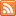# Content Tagged “LO 6.13”## Unit 3B: Random Variables

Published: Aug 27th, 2012

Introduction Random Variables Types of Random Variables CO-6: Apply basic concepts of probability, random variation, and commonly used statistical probability distributions. Video: Unit 3B Random Variables (10:00) Introduction In the remaining sections in […]